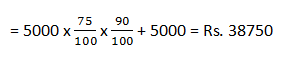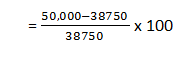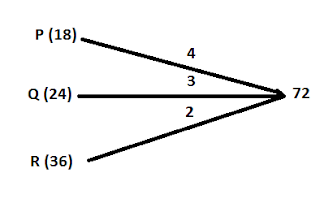New Student User - Use Code HELLO

# Related Quantitative Aptitude Questions for IBPS Clerk Prelims

1. Each Student of a class collected some money for the trip. The total money collected by each student was equal to the cube of the total number of students. If the total amount collected was Rs. 29791. find the total number of students.
1. 31
2. 30
3. 28
4. 37
5. 39

2. A dealer buys an article marked at Rs. 50,000 at 25% and 10% successive discount. He spends Rs. 5000 on its repair and sells it for Rs. 50,000. What is his loss or gain percentage?
1. 33.63% loss
2. 26.43% gain
3. 1.25% gain
4. 29.03%
5. 27.13% loss

3. The average age of four boys, five years ago was 9 of all the five is 15 years. The present age of the new boy is
1. 22 years
2. 24 years
3. 21 years
4. 18 years
5. 19 years

4. P, Q and R can do a piece of work in 18,24 and 36 days on the second day and P on the third day, again R on the fourth day and so on. Then in how, many days will the work be completed?
1. 25
2. 24
3. 22
4. 20
5. 26

5. Harshika wants to purchase a mobile handset. The shopkeepers told her to pay 30% tax if she asked for the actual sale price (without tax) of the mobile and paid to the shopkeeper Rs. 16150. In the doing so, she managed to avoid to pay the 30% tax. What is the amount of discount that she received on the selling price (inclusive of tax)?
1. Rs. 5495
2. Rs. 5850
3. Rs. 5950
4. Rs. 5685
5. Rs. 5975

6. The diluted wine contains only 10 litres of wine and the rest is water. A new mixture is formed by replacing mixture with the pure wine whose concentration is 40%. How many litres of mixtures was replaced if initially there were 40 litres of water in the mixture?
1. 10 litre
2. 12 litre
3. 9 litre
4. 11.5 litre
5. 12.5 litre

7. A tank can be filled by a tap in 6 hours and emptied by an outlet pipe in 8 hours. How long will it take to fill the cistern if both the taps are opened together?
1. 24 hours
2. 22 hours
3. 21 hours
4. 18 hours
5. 20 hours

1. 1;
Total number of students2. 4;
CP of the article∴ Profit percentage= 29.03% gain

3. 5;
Five years ago the average age of 4 boys = 9 years
The present average age of 4 boys = 9+5 = 14
So the present age of new boys = 15 + 4 x 1 = 19 years

4. 2;Units of work done in 3 days = (2 + 3 + 4) = 9
∴ 72 units of work will be done in = 3 x 8 = 24 days

5. 3;
SP = 100, SP (with tax) = 130
New SP = 100 - 5 = 95
Effective discount = 130 - 95 = 35
So, when SP of 95, discount = 35
And when SP of Rs. 16,150, discount = 35/95x16150 = Rs. 5950

6. 5;
Initial ratio of water to wine = 40 : 10 = 4: 1
Final ratio of water to wine = (4 : 1) = 24:6
Required, final ratio of water to wine
= 60 : 40
= (6 : 4)
= 24 : 16x⁴
If total mixture is 40 litres, mixture replaced is = 10 litres
Here total mixture is 50 litre, mixture replaced is = 12.5 litres

7. 1;Total time required to fill the tank = 24/4-3 = 24 hours